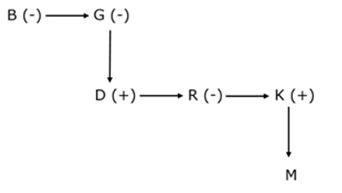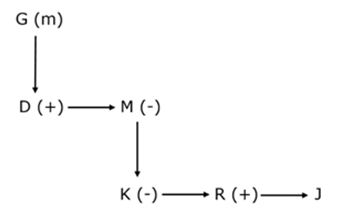# SBI Clerk Mains Reasoning (Day-12)

Dear Aspirants, Our IBPS Guide team is providing new series of Reasoning Questions for SBI Clerk Mains 2020 so the aspirants can practice it on a daily basis. These questions are framed by our skilled experts after understanding your needs thoroughly. Aspirants can practice these new series questions daily to familiarize with the exact exam pattern and make your preparation effective.

Start Quiz

Ensure Your Ability Before the Exam – Take SBI Clerk 2020 Mains Free Mock Test

Number series condition based

Direction (1 – 3): In each of the following questions, two rows of numbers are given. The resultant number in each row is to be worked out separately based on the following rules and the questions below the rows of numbers are to be answered. The operations of numbers progress from the left to the right.

Rules:
(i) If an even number is followed by another even number they are to be added.

(ii) If an even number is followed by a prime number they are to be multiplied

(iii) If an odd number is followed by an even number, the even number is to be subtracted from the odd number

(iv) If an odd number is followed by another odd number the first number is to be added to the square of the second number

(v) If an even number is followed by a composite odd number, the even number is to be divided by the odd number.

1) What is the half of the sum of the resultants of the two rows?

I. 54 27  3

II. 7  3  12

a) 17

b) 15

c) 19

d) 13

e) None of the Above

2) What is the product of the resultants of the two rows?

I. 25   12   8

II. 18  18   9

a) 16

b) 18

c) 20

d) 24

e) None of the Above

3) what is the difference between the resultants of the second row and first row?

I.  12    7  16
II. 67  12   3

a) 36

b) 34

c) 32

d) 24

e) None of the Above

Seating:

Direction (4 – 8): Answer the questions on the basis of the information given below.

Eight people A, B, C, D, E, F, G and H are sitting around a circular table with equal distance between each other but not necessarily in the same order. Some of them are facing the centre while some are facing outside. (I.e. away from the centre) B sits third to the left of A. E sits to the immediate right of H. D faces a direction opposite to that of B. Immediate neighbours of E face opposite directions(i.e. if one neighbour faces the centre then the other neighbour faces outside and vice-versa.) Only one person sit between E and D.G sits second to the right of D. Both G and C face the same direction as H. (i.e. if H faces the centre then G and C also faces the centre and vice-versa.) Only three people sit between B and H. E does not face outside. Immediate neighbours of F faces opposite directions (i.e. if one neighbour faces the centre then the other neighbour faces outside and Vice-versa.)

4) Which of the following statements is true as per the given arrangement?

a) F faces the centre

b) Only three people sit between E and A

c) G sits to the immediate right of C

d) None of the given options is true

e) C is an immediate neighbour of D

5) Who amongst the following sits third to the left of E?

a) F

b) C

c) B

d) A

e) G

6) How many people face the centre as per the given arrangement?

a) Four

b) One

c) Two

d) Three

e) More than four

7) What will come in place of question mark (?) in the given series based on the positions as given in the arrangement?
EH AG BE HB ?

a) DC

b) HD

c) CF

d) DA

e) AH

8) As per the given arrangement D is related to A in the same way as B is related to H, Following the same pattern to who is C related?

a) F

b) E

c) N

d) G

e) P

Blood relation coded type:

(Directions 9–10): Study the following information carefully and answer the questions given below it.

‘A @ B’ means ‘A is father of B’.

‘A % B’ means ‘A is brother of B’.

‘A # B’ means ‘A is mother of B’.

‘A & B’ means ‘A is sister of B’.

9) In given statement “B&G#D%R&[email protected]”, how G is related to M?

a) Aunty

b) Maternal Grandfather

c) Father-in-law

d) Grandmother

e) Maternal Aunty

10) Which of the following option correctly fill in the blanks in given statement below such that D is maternal uncle of J?

[email protected]%M__K__R%J

a) &, @

b) @, %

c) #, &

d) &, %

e) #, @

Directions (1-3):

54 / 27 = 2 [Rule v] 2 * 3 = 6 [Rule ii] R1 = 6

7 + (3)² = 16 [Rule iv] 16 + 12 = 28 [Rule i] R2 = 28

34 / 2 = 17

25 – 12 = 13 [Rule iii] 13 – 8 = 5 [Rule iii] R1 = 5

18 + 18 = 36 [Rule i] 36 / 9 = 4 [Rule v] R2 = 4

= 5 * 4 = 20

12 * 7 = 84 [Rule ii] 84 + 16 = 100 [Rule i] R1 = 100

67 – 12 = 55 [Rule iii] 55 + (3)² = 64 [Rule iv] R2 = 64

100 – 64 = 36

Direction (4 -8):B sits third to the left of A. Only three people sit between B and H.E sits to the immediate right of H. Immediate neighbours of E face opposite directions. Only one person sit between E and D. G sits second to the right of D.Both G and C face the same direction as H. E does not face outside. D faces a direction opposite to that of B. Immediate neighbours of F faces opposite directionsDirections (9-10):

We have:

B&G#D%R&[email protected]

From above given information we have:Clearly, G is grandmother of M.

Hence, option D is correct choice.

We have:

[email protected]%M__K__R%J

Clearly, option C satisfies given condition and after applying we get:Check Here to View SBI Clerk Mains 2020 Reasoning Ability Questions Day – 11 Day – 10 Day – 09 Click Here for SBI Clerk 2020 – Detailed Exam Notification